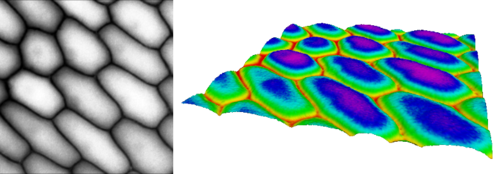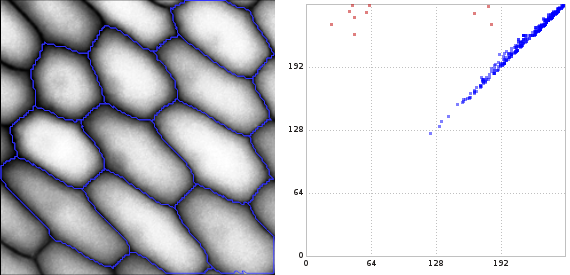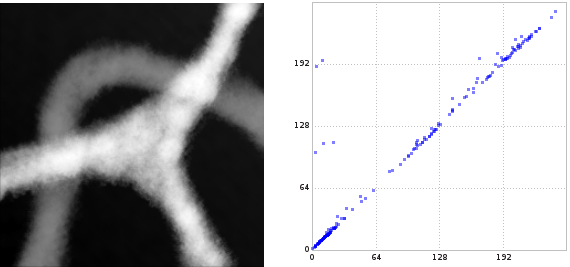# libstick: Demonstration

The following demonstration refers to the current version 0.2 of libstick. (Version 0.1 had no concept of a simplicial_function, but incorporated it into simplicial_complex.)

## From images to complexes

Persistent homology has many applications, one of them is image analysis. Consider the following image of onion cells from an epidermal peel1. On the left we see the gray-scale image and on the right we see the image interpreted as gray-value function $$\Omega \to [0,255]$$ over the rectangular image domain $$\Omega \subset \mathbb{R}^2$$.Using libstick, one can convert an image to a simplicial function on a simplicial complex in the following sense:

• Simplicial complex. Each pixel gives rise to a vertex (0-simplex) and any two pixels sharing an edge give rise to an edge (1-simplex) between the corresponding vertices representing the pixels. Each of the square faces is split into triangles by an additional 1-simplex and all the triangles are inserted as 2-simplices.

• Simplicial function. Each simplex is assigned a function value as follows: Each vertex gets assigned the gray value (between 0 and 255) of its pixel and all edges and triangles get as value the maximum value of the incident vertices.

Next, one creates a monotone filtration from the simplicial complex:

Finally, we run the matrix reduction algorithm and build the persistence diagrams. This allows us to ask, for instance, for the persistent Betti-1 number in the interval [96, 192]. The function interpret_reduction() prints information on the sequence of insertions of simplices and which cycles are born or killed due to these insertions. The function interpret_persistence() prints the points in the persistence diagram with their birth and, if existent, death times.

## Finding cycles in images

In the figure below, we see on the right the 1-dimensional persistence diagram computed by libstick and visualized by lyxtor2. The red points in the diagram have been selected and visualized as blue cycles on the left.3 Seven of them are born in the interval [16, 64] and three of them are born in [160, 192]. (The latter three correspond to those onion cells that are a bit trimmed by the image boundaries.) The birth time of a cycle is the gray value of the brightest pixel of a cycle. The death time of a cycle is the gray value of the brightest pixel enclosed by it.## Merging components

Below we see another gray-scale picture and the 0-dimensional persistence diagram of the sub-level set filtration. The picture shows a very bright star shape with three branches and a mid-gray path crossing two of the bright branches. The 0-dimensional persistence diagram reflects this: At a low level, we have 6 quite black components. As the level rises beyond the gray value of the mid-gray path, 3 components are merged with 3 others, causing three points in the diagram at about a gray value of 100. Later, when the level rises beyond the gray value of the star shape, the final three components are merged to a single one, causing the two points in the diagram at a gray value of about 192.1. Original picture published by Adrian J. Hunger at Wikipedia under CC BY-SA 3.0 license. Preprocessed in RawTherapee and lyxtor2. The modified versions published here are therefore again licensed under CC BY-SA 3.0

2. Lyxtor is a software I wrote at IST Austria that analyzes lymph vessel networks using, in particular, persistent homology computed by libstick.  2

3. The cycles are retrieved from the transformation matrix. However, a different matrix reduction algorithm may produce a different transformation matrix. That is, the cycles should be seen as example elements of a (homology) equivalence class of cycles. The reduced boundary matrix (and, thus, the persistence diagram) are unique; they do not depend on details of the algorithm.